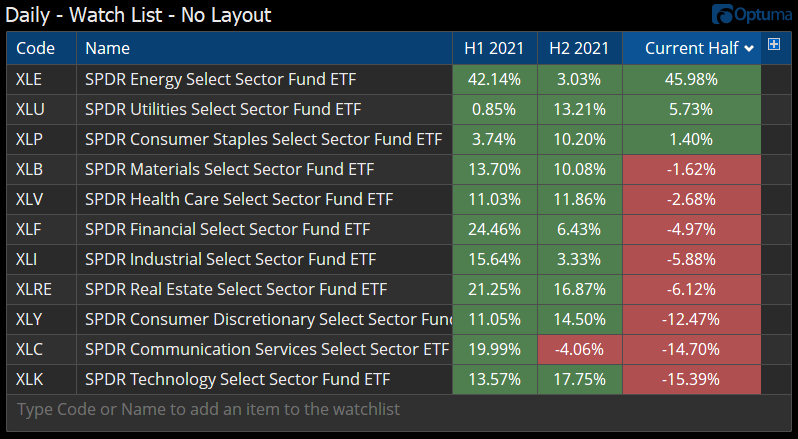Select Page

# Reply To: Half function idea

Optuma Forums Optuma Feature Requests Half function idea Reply To: Half function idea

#67989

Thanks Jeff. You could adapt the formulas used to calculate the Quarterly performance seen here. For example, H1 2021 would be as follows:

For H2 2021 change  M1 to MONTHNUM() == 12 and YEARNUM() == 2021; and M2 to MONTHNUM() == 6 and YEARNUM() == 2021;

The Current Half would be M1=CLOSE(); and M2 = MONTHNUM() == 12 and YEARNUM() == 2021; before the end of June, and then M2 = MONTHNUM() == 6 and YEARNUM() == 2022; afterwards.

Here’s the SPDR ETFs (workbook attached):